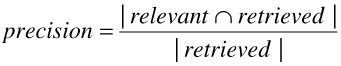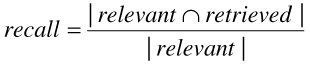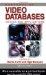# 7. Evaluating Relevance Feedback

## 7. Evaluating Relevance Feedback

There are two aspects to evaluating how good a retrieval system performs its tasks: quantitatively and qualitatively. The quantitative approach to evaluating a system focuses on execution speed: the time it takes the retrieval system to perform a search and return the results to the user. Performance in this sense is of chief importance for an interactive retrieval system and has been extensively studied for many types of search technology including Databases  and Information Retrieval systems  and is also discussed in other chapters of this book. But when relevance feedback changes the interpretation of a query and a new set of answers is computed, it is often possible to utilize the work already performed for the old query to answer the new query. Work in this area has been done for multimedia retrieval  as well as for database search . We do not further discuss techniques for efficient evaluation of new queries; instead we discuss the relevant issues in evaluating the quality of retrieval.

With conventional exact search tools as varied as the UNIX grep utility and SQL databases there is no need to evaluate the quality of the answers since a value either satisfies or does not satisfy the search criteria. A system that returns wrong answers is clearly identifiable and undesirable. But under the similarity search paradigm, answers are given based on how closely they match a user's query and preferences; there is no clear-cut way to distinguish correct answers from wrong answers. Therefore the issue of quality in the results is of prime importance when evaluating a retrieval system.

Since relevance feedback seeks to improve the quality of retrieval, it becomes important to quantify the gains made from iteration to iteration.

We discuss two approaches to evaluate the quality of retrieval results: (1) precision and recall, and (2) normalized recall.

Text retrieval systems typically follow the very popular precision and recall  metrics to measure the retrieval performance. Precision and recall are based on the notion that for each query, the collection can be partitioned into two subsets of documents. One subset is the set of relevant documents and is based on the user's criteria for relevance to the query. The second is the set of documents actually retrieved by the system as the result of the query. Precision and recall are defined as follows:

• Precision is the ratio of the number of relevant images retrieved to the total number of images retrieved. Perfect precision (100%) means that all retrieved images are relevant. Precision is defined as:• Recall is the ratio of the number of relevant images retrieved to the total number of relevant images. Perfect recall (100%) can be obtained by retrieving the entire collection, but at a cost in precision. Recall is defined as:The retrieval system is characterized by constructing a precision-recall graph for each relevance feedback iteration in a query. The precision-recall graph is constructed by incrementally increasing the size of the retrieved set, that is, by iteratively retrieving the next best result and measuring the precision every time a relevant answer is found (i.e., at different values of recall). Usually, the larger the retrieved set, the higher the recall and the lower the precision become. Figure 21.7 shows an example precision recall graph for the original query and 2 iterations of relevance feedback.Figure 21.7: Sample Precision-Recall Graph

A problem with the precision and recall approach is that it ignores the ordering among relevant results. If we interpret the improvement derived from relevance feedback to include the ordering among relevant results, then we must find a measure that captures this ordering. The query result after each iteration is a list of objects ranked on how closely each object matches the query. The normalized recall metric  is designed to compare ranked lists with special consideration for the ordering.

The normalized recall metric compares two ranked lists, one of relevant items (the ground truth) and one of retrieved items (the result of a query iteration):where rank(List,o) is the rank of object o in List. The metric computes the rank difference of the two lists and normalizes the result by the highest possible rank difference, thus producing a value in the range 0 (worst) to 1 (best). The metric is sensitive to the position of objects in the ranked list. This sensitivity to rank position is suitable for measuring the effectiveness of the relevance feedback process by comparing the relevant list to the result across feedback iterations. As the lists converge, the metric results in a better value. The normalized recall metric is meant to compare a list of relevant items to the fully ranked list of all objects in a database. The problems with this metric are the following:

• A few poorly ranked, but relevant, items have a great impact because a poorly ranked item contributes a large value of rank difference. In practice, the results seen by the user are much less than the database size.

• The database size has a great impact on the metric. In a large database, only a small part of it is actually explored by the user; the rest is of no use. Therefore, using the database size as an important part of the denominator in the equation obscures the difference of two good retrieved lists because the large denominator dilutes the rank difference significantly.

• Each ranked item is equally important. That is, the best relevant item is as important as the worst relevant, but still relevant, item.Handbook of Video Databases: Design and Applications (Internet and Communications)
ISBN: 084937006X
EAN: 2147483647
Year: 2003
Pages: 393